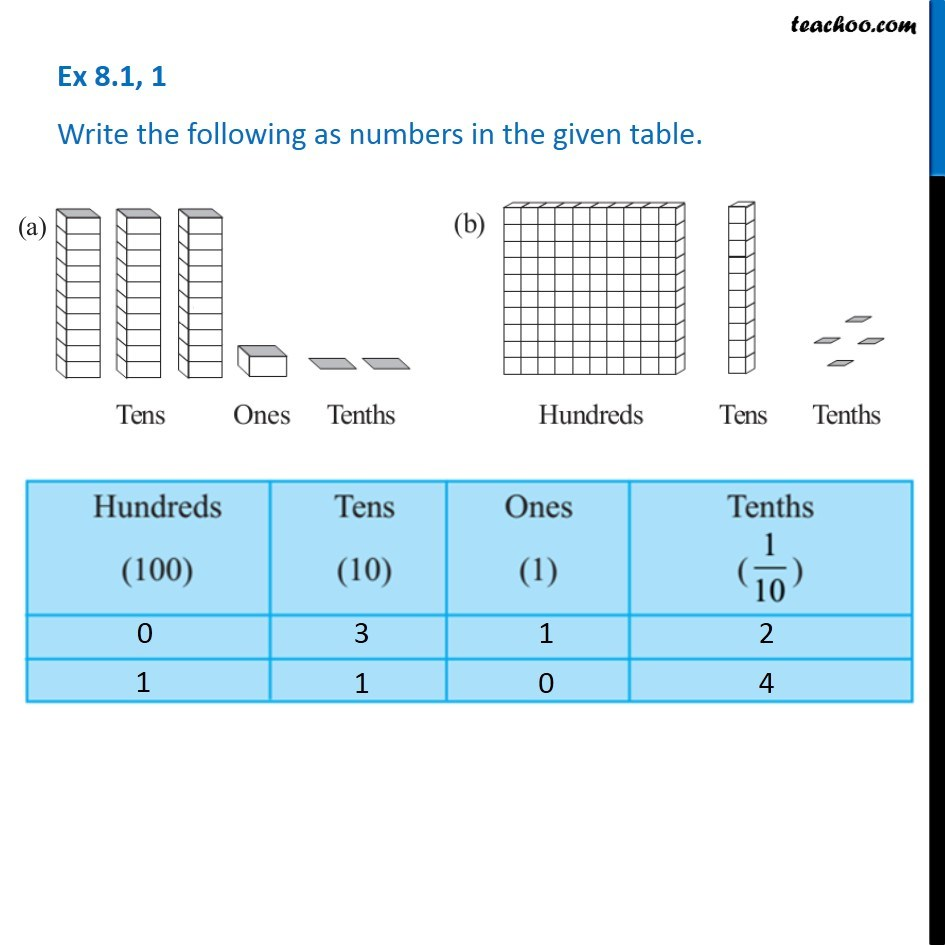Tenth

Chapter 8 Class 6 Decimals
Concept wiseIntroducing your new favourite teacher - Teachoo Black, at only ₹83 per month

### Transcript

Ex 8.1, 1 - Chapter 8 Class 6 Integers (NCERT Book) Write the following as numbers in the given table. For (a) Hundreds (100) = 0 Tens (10) = 3 Ones (1) = 1 Tenths (1/10) = 2 For (b) Hundreds (100) = 1 Tens (10) = 1 Ones (1) = 0 Tenths (1/10) = 4